Power of a Quotient

When the elements of a quotient have exponents and the quotient is raised to a power, then the power multiplies the exponent of each element in the quotient.

Power of a Quotient Rule :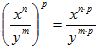.

Example 1

Simplify the expression: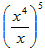.

Step 1. Use the Power of a Quotient Rule.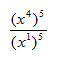Step 2. Use the Power of a Power Rule to simplify the resulting expression.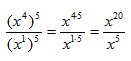Step 3. If the bases are the same, use the Quotient of a Power Rule for Exponents to simplify the resulting expression.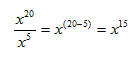Listen